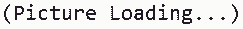You've successfully subscribed to The Finbox Blog
Welcome back! You've successfully signed in.# Capital Asset Pricing Model (CAPM) Explained

### What is CAPM (Capital Asset Pricing Model)?

The Capital Asset Pricing Model (CAPM) is a model used to calculate the cost of equity for a company based on its risk, represented by its stock's beta. From an investor’s perspective, CAPM is used to calculate the expected return of a stock investment. According to this theory, the only way an investor can earn above-average investment returns is to invest in riskier securities.

In short, CAPM is a model that is used in the financial sector to help describe the relationship between the expected risks and returns of an investment. While many investors raise doubts about the CAPM model, it is still widely used in the finance sector.### CAPM Formula Explained

According to the Capital Asset Pricing Model (CAPM), a company's cost of equity is equal to:

Cost Of Equity = Rrf + [β x (Re – Rrf)]

Where:

• Rrf = Risk-free rate
• β = Stock's beta
• Re = Stock Market Expected Return
• (Re - Rrf) = Equity Risk Premium

Let's break down each of the formula's components:

• Risk-Free Rate: The risk-free rate is equal to the 10-year government note yield of the company's operating country. For example, if we want to calculate Netflix's cost of equity, we should take the 10-year US treasury note yield as the risk-free rate.
• Beta (β): The βeta is a financial metric that measures the volatility of a stock's price changes compared to the market. According to the CAPM, higher βeta equals higher risks. However, many investors disagree with this risk-valuation approach because an investment risk should be evaluated based on a company's fundamentals rather than its stock's price movements.
• Equity Risk Premium: The equity risk premium represents the stock market's additional return relative to the 10-year Government note. Market returns and treasury rates can fluctuate significantly in different periods, resulting in different equity risk premiums. Thus, investors calculate the equity risk premium with the methodology they find most appropriate. Here at Finbox, we calculate the equity risk premium using the Duff & Phelps methodology.### CAPM Example Calculation—Netflix's Cost Of Equity

To illustrate, we can calculate Netflix (NASDAQGS:NFLX)'s cost of equity using the CAPM formula. With the Finbox data explorer, we can find the values of the metrics required to apply the formula:

Here's Netflix's cost of equity (expected return) calculation using the CAPM formula:

Cost Of Equity = Risk free rate + Beta*Equity Premium
Cost Of Equity/Expected Return = 0.70% + 0.97*5.5% = 6.03%

### CAPM Calculator - Free Excel Template

You can calculate CAPM and WACC for every single company in the world using this template. You just have to enter the required data of the company whose ratio you want to calculate, and the model will do everything automatically.

You can use the Finbox Data Explorer to get all the data you need for 100,000+ companies worldwide.

Here's a preview of Finbox's CAPM Calculator:Although the Capital Asset Pricing Model is widely used in financial modeling to evaluate the intrinsic value of a business, it has some serious drawbacks. Like most theoretical models, CAPM doesn't actually reflect reality. So let's discuss some of its disadvantages:

1# βeta Is Not An Objective Metric

As discussed above, a stock's beta is equal to the volatility of its price changes compared to the market. If we select different periods, we'll get different betas, resulting in an inconsistent investment risk valuation.

For example, here are Apple's βeta for different periods:

• Apple's βeta (5-Year) = 1.31
• Apple's βeta (2-Year) = 1.02
• Apple's βeta (1-Year) = 0.98

As you can observe, two analysts using different βetas will obtain different results.

2# Volatility Isn't Risk

One of the Capital Asset Pricing Model's main assumptions is that a stock's volatility represents the investment risk. But this statistical approach often doesn't reflect reality. Let's consider Apple (NASDAQGS:AAPL)'s stock as an example.As depicted above, Apple is one of the few outperformers of the COVID-19 pandemic. Its stock price skyrocketed at the expense of its valuation metrics, resulting in a tremendously overpriced stock. A more expensive stock usually equals a riskier investment, right?

Nevertheless, Apple's 1-year Beta is much lower than the corresponding 5-year metric. So, according to the Capital Asset Pricing Model, Apple's stock is now less risky than the past. Of course, Apple's valuation tells a completely different story.

Tags